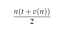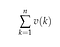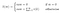# I’m overthinking this programming Q-and-A business

## A simple fix, or a way to make it right?

For many years I was active in a couple of programming Q-and-A communities. It was very rewarding since many questions became a prompt for me to learn more about a problem or language feature before I answered. What was a bit tedious in the long run, apart from the fact that those frequently asked questions are asked very frequently, was that so few questioners seemed interested in learning more about whatever it was that stumped them. Mostly, they wanted someone to find an error for them so that they could go on in the same way, producing similar errors again and again.

As an example, someone posted this:

What is the mistake in the following code:

int count = 1, sum = 0;
do {sum = sum + count;} while (count <= 10);

Well, the mistake is that the variable count is never incremented, which means that the loop never ends (it has the value 1 and will never be larger than that). Here you go:

`int count = 1, sum = 0;do {sum = sum + count; ++count;} while (count <= 10);`

This is one possible kind of answer: an ad-hoc minimal rewrite. It’s a straightforward answer without unnecessary noise.

We found the error by observing that the program did not terminate, which suggested that the loop statement was wrong. By rule of thumb, the condition to a conditional loop is the first place to look: if it depends on a variable, the loop must modify that variable correctly or there will be problems. In this case, the error wasn’t one of the dozens of subtle errors that can escape notice for a long time.

The error occurred because the loop statement is a bit complex and the programmer wasn’t paying attention. If things can go wrong, they will. If we keep producing code without any real method, the correctness of the code will be hit-or-miss, and we are going to have to stop and fix problems like this again and again. Good programming practice involves leaving as little opportunity as possible for errors to sneak in.

The poster of the question was probably trying out the do-while statement, but I’ll discuss this as if the goal was to calculate an arithmetic series correctly.

An arithmetic sequence is described by the parameters n (number of elements), t (starting point, i.e. value of first element), and d (summand, i.e. the difference between each element and the preceding element). These parameters can be used to define a function v. Given an element index k in the range 1≤kn and the formula t+d(k-1), v(k) gives the value of each element. By definition, v(1)=t.

The corresponding arithmetic series can be calculated using n and v either non-iteratively asThe series as the product of the number of elements and the arithmetic mean of the sum of the first and last elements.

or iteratively asThe series as the sum of v(k) for k=1 to n.

By these definitions, we can calculate the series without using a loop. The following is a simple translation of the non-iterative formula into C source code. The parameters n, t, and d are collected in a record structure which both the v-function and the main procedure use freely. In a more sophisticated program, the parameters could be variables, function arguments, or other mechanism for managing access to the values.

This code does not have the mistake in the example, simply because there is no loop. It’s a simple formula calculation, and it works for all arithmetic series where the number of elements, the value of the first element, and the difference between elements is known. Simplicity and clarity are good for bug prevention.

We should be able to safely translate the second formula for calculating the series to a loop if we study the formula carefully and translate step by step.

The summation formula corresponds to this algorithm fragment, which leaves the series in the variable sum:

This translates directly to the following C source:

The definitions in the product example should be included to complete the program.

By carefully translating the structure in the solution to source code, many bugs can be prevented. This kind of pattern is something the beginning programmer should try to identify, practice, and have ready to use.

We can also write small, reliable pieces of code by using methods that establish correctness. I am basing my description here on Hoare’s “An Axiomatic Basis for Computer Programming” (and I’m using the old-style notation instead of the later {P} Q {R} notation).

The general method is to set up triples like this:

P and R are assertions — expressions that must be true if the program is to be considered correct. Q represents some amount of code, and the formula means: “if P is true, and Q is executed, R must be true”. The assertion to the left is called the precondition, and the one on the right the postcondition.

First, a predicate that will be used to check the correctness of the series-building code:A predicate S(m) that inspects the variable sum and compares its value to the expected value.

I.e. S(0) is true if sum is 0, and S(m) is true if sum is the sum from v(1) to v(m). The whole procedure is correct if the precondition S(0) and the postcondition S(n) hold.

If we check the first formula for correctness:

The precondition is true, because we set sum to 0 and then test if the value is 0. When the code has been executed, the value in sum is equal to the sum from v(1) to v(n), which makes the postcondition true. The code is correct.

We can also use this method to build an iterative solution. For the base case, we will write correct code that brings us from S(0) to S(1):

This says that when we’ve added the first element to the empty sum, sum holds the value of the first element. Seems about right. The general case has the same form as the base case:

This says that when we are at element k, we go from S(k-1) being true, i.e. sum has the total of elements 1 to k-1. After running the code, we are at S(k), because another element has been added.

To be able to compose a number of these formulas to an iteration, we need to make the precondition and the postcondition the same expression. We can do that by first setting up the incrementation of the k value:

This says that if we have the sum of k elements, incrementing k means that the now have the sum of k-1 elements.

The matching assertions S(k) means that we can compose these parts, and as this aggregate also has matching assertions S(k-1), it can be composed with itself in an iteration. This conversion means that a term needs to be added to each of the assertions: k = 1 to the precondition, and k = n + 1 to the postcondition.

Now, asserting k=1 and S(k-1) together means that we are asserting S(0). Likewise, asserting k = n + 1 and S(k-1) together means asserting S(n). We now have a program that has been proven to do the calculation we wanted. We leave out the assertions and move sum and k from definitions into the formula:

Which is the same as:

Which is translated to C in the above.

Algorithmician, history buff, non-practicing hedonist. Whovian, ghiblist: let there be wonder. Argumentative, punster, has delusions of eloquence.

## More from Peter Lewerin

Algorithmician, history buff, non-practicing hedonist. Whovian, ghiblist: let there be wonder. Argumentative, punster, has delusions of eloquence.